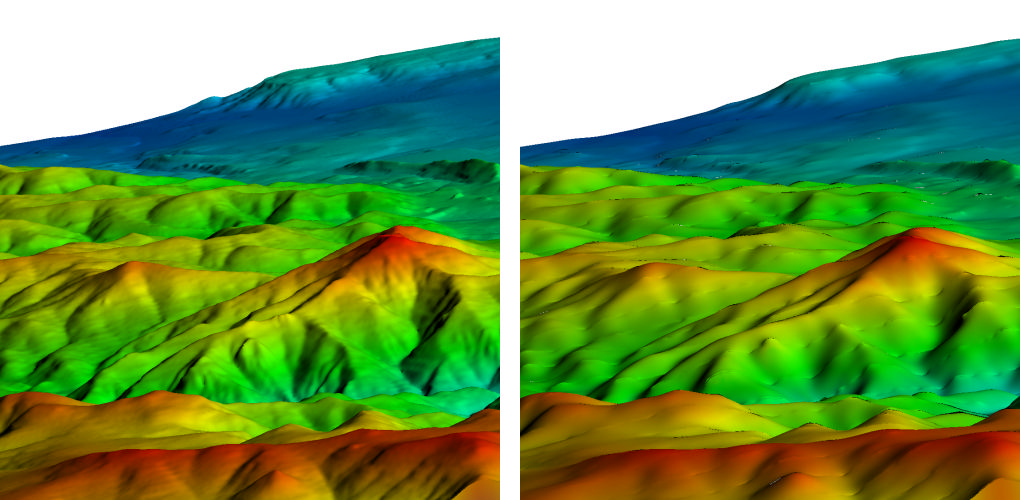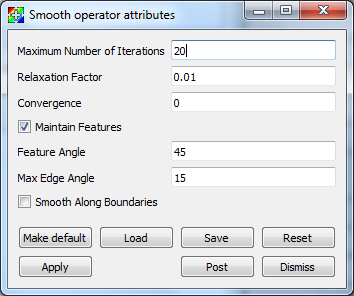# 4.3.29. Smooth operator¶

The Smooth Operator applies Laplacian Smoothing to a mesh, making the cells better shaped and the nodes more evenly distributed, which results in smoother edges and peaks throughout the mesh. Each node is moved towards a coordinate that is the average of its connected nodes. The relaxation factor determines how far along that path to move the node. A sweep over all nodes is a single iteration.Fig. 4.71 Smooth operator example

## 4.3.29.1. Using the Smooth operator¶

The Smooth operator has a number of controls that can be used to tune mesh smoothness.

Maximum number of iterations

Controls the maximum number of times the mesh relaxation algorithm is applied to the input mesh. Larger numbers of iterations will produce smoother meshes but will also take more time to compute. Values must be integers.

Relaxation Factor

Controls how much the mesh is relaxed. Values near 1 produce a mesh that is very smooth relative to the input mesh. Values must be floating point numbers between 0 and 1.

Convergence

Limit the maximum point motion. The smoothing process will terminate if the maximum point motion during an iteration is less than this value. Smaller numbers result in more iterations, and if 0 is supplied then the Smooth operator will perform Maximum number of iterations. Values must be floating point numbers between 0 and 1.

Maintain Features

When the surface angle between two zones sharing an edge is greater than Feature Angle, then the edge is classified as a feature edge. The nodes in the mesh are then classified as simple (not used by a feature edge), interior edge (used by exactly two feature edges), or fixed (all other nodes). Simple nodes are smoothed as before, fixed nodes are not smoothed at all, and interior edge nodes are only smoothed along the two connected feature edges, and only if the angle between the edges is less than Max Angle Edge. This distinction allows the smoothing operation to preserve sharp peaks in the mesh while still smoothing out most of the mesh.

Feature Angle

Used to determine the number of feature edges for each node. Values must be floating point numbers between 0 and 90.

Max Angle Edge

Used to determine if interior edge nodes will be smoothed. Values must be floating point numbers between 0 and 90.

Smooth Along Boundaries

Enable the smoothing operation on nodes that are along the boundaries of the mesh.Fig. 4.72 Smooth attributes